Bandit问题是强化学习中一类重要的问题，由于它定义简洁且有大量的理论分析，因此被广泛应用于新闻推荐，医学试验等实际场景中。随着人类进入大数据时代，用户对自身数据的隐私性日益重视，这对机器学习算法的设计提出了新的挑战。 为了在保护隐私的情况下解决 Bandit 这一经典问题， 北京大学和华为诺亚方舟实验室联合提出了 基于本地差分隐私的 Bandit 算法 ，论文已被 NeurIPS 2020 录用， 代码已基于 MindSpore 开源首发 。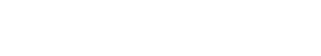Bandit 问题有 context-free 和 contextual 两种常见的设定，下面给出它们具体的数学定义。

## 【Context-Free Bandit】## 【Contextual Bandit】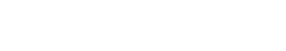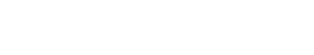## Context-Free Bandit## Contextual Bandit【定理】 依照至少为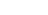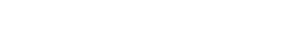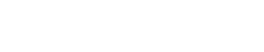MovieLens 是一个包含多个用户对多部电影评分的公开数据集，我们可以用它来模拟电影推荐。我们通过src/dataset.py 来构建环境：我们从数据集中抽取一部分有电影评分数据的用户，然后将评分矩阵通过 SVD 分解来补全评分数据，并将分数归一化到[−1,+1]。在每次交互的时候，系统随机抽取一个用户，推荐算法获得特征，并选择一部电影进行推荐，MovieLensEnv会在打分矩阵中查询该用户对电影对评分并返回，从而模拟用户给电影打分。

class MovieLensEnv:    def observation(self):        """random select a user and return its feature."""        sampled_user = random.randint(0, self._data_matrix.shape - 1)        self._current_user = sampled_user        return Tensor(self._feature[sampled_user])    def current_rewards(self):        """rewards for current user."""        return Tensor(self._approx_ratings_matrix[self._current_user])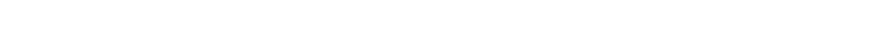import mindspore.nn as nn
class LinUCB(nn.Cell):    def __init__(self, context_dim, epsilon=100, delta=0.1, alpha=0.1, T=1e5):    ...        # Parameters        self._V = Tensor(np.zeros((context_dim, context_dim), dtype=np.float32))        self._u = Tensor(np.zeros((context_dim,), dtype=np.float32))        self._theta = Tensor(np.zeros((context_dim,), dtype=np.float32))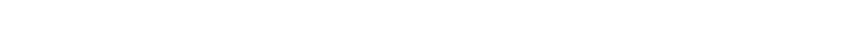import mindspore.nn as nn
class LinUCB(nn.Cell):...    def construct(self, x, rewards):        """compute the perturbed gradients for parameters."""        # Choose optimal action        x_transpose = self.transpose(x, (1, 0))        scores_a = self.squeeze(self.matmul(x, self.expand_dims(self._theta, 1)))        scores_b = x_transpose * self.matmul(self._Vc_inv, x_transpose)        scores_b = self.reduce_sum(scores_b, 0)        scores = scores_a + self._beta * scores_b        max_a = self.argmax(scores)        xa = x[max_a]        xaxat = self.matmul(self.expand_dims(xa, -1), self.expand_dims(xa, 0))        y = rewards[max_a]        y_max = self.reduce_max(rewards)        y_diff = y_max - y        self._current_regret = float(y_diff.asnumpy())        self._regret += self._current_regret
# Prepare noise        B = np.random.normal(0, self._sigma, size=xaxat.shape)        B = np.triu(B)        B += B.transpose() - np.diag(B.diagonal())        B = Tensor(B.astype(np.float32))        Xi = np.random.normal(0, self._sigma, size=xa.shape)        Xi = Tensor(Xi.astype(np.float32))
# Add noise and update parameters        return xaxat + B, xa * y + Xi, max_a

import mindspore.nn as nn
class LinUCB(nn.Cell):...    def server_update(self, xaxat, xay):        """update parameters with perturbed gradients."""        self._V += xaxat        self._u += xay        self.inverse_matrix()        theta = self.matmul(self._Vc_inv, self.expand_dims(self._u, 1))        self._theta = self.squeeze(theta)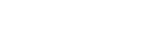x 轴：交互轮数
y 轴：累积 regret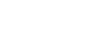1. Kai Zheng, Tianle Cai, Weiran Huang, Zhenguo Li, Liwei Wang. “Locally Differentially Private (Contextual) Bandits Learning.” Advances in Neural Information Processing Systems . 2020.

2. LDP LinUCB 代码：

https://gitee.com/mindspore/mindspore/tree/master/model_zoo/research/rl/ldp_linucb